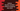# Python program to declare variables without assigning any value## Python program to declare variables without assigning any value:

In python, we can declare variables without assigning any value to it. Whenever we create one variable in Python, we assign one value to it and python automatically defines its type.

For example :

``````my_value = 10
print(type(my_value))

my_value = 'Hello'
print(type(my_value))``````

In this example, I have declared one variable my_value with value 10. Next, I assigned ‘Hello’ to this variable. For both cases, we are printing its type. It will give the below output:

``````<class 'int'>
<class 'str'>``````

So, the type of this variable is changed once we change its value.

### Declaring a variable without assigning any value:

In python, we can assign None to a variable that will declare its value without any value. If we want, we can assign any value to it later.

``````my_value = None

print(type(my_value))``````

This program will print:

``<class 'NoneType'>``

If you assign any value to this variable, it will change the type :

``````my_value = None
print(type(my_value))

my_value = 10
print(type(my_value))``````

It prints :

``````<class 'NoneType'>
<class 'int'>``````